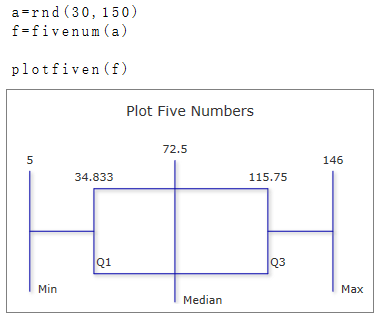# PlotFiveN Function

Visualizes the five most important statistical values

### >Description

The function $$PlotFiveN$$ creates a graphical summary of the five most important statistical values from a set of data. To display the graphic, a plot box must be opened.

The argument must be a list containing the five values. The list can be generated from the statistical data using the FiveNum function.

The following values are displayed: Minimum, First Quartile, Middle Value, Third Quartile, and Maximum Value.

### Syntax

Plotfiven (n,)

Plotfiven (n, id)

### Parameter

n

A list containing the five values to be displayed.

### Optional Parameter

id

Id number of the plot box, if more than one box is open on the worksheet.

### Beispiel